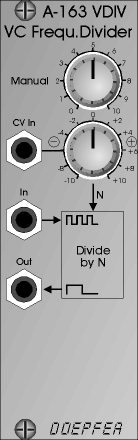﻿ A-163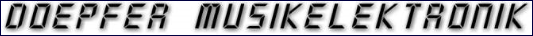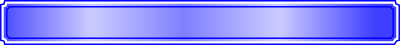Analogue  Modular  System  A-100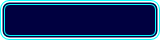WidthHPDepth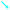mmCurrentmA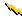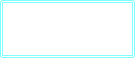Owners ManualVoltage Controlled Frequency Divider

The A-163 is a voltage controlled audio frequency divider. The frequency of the input signal (usually the rectangle output of a VCO) is divided by an integer factor N (N = 1, 2, 3, 4 ... up to about 20). N can be adjusted manually and modulated with an external control voltage (e.g. from LFO, ADSR, Random, MIDI-to-CV, Theremin, Light-to-CV, analogue sequencer) with attenuator.

The output waveform is rectangle with 50% duty cycle. Unlike the A-115 with fixed dividing factors (2, 4, 8, 16) the dividing factor of the A-163 is voltage controlled and can be any integer value between 2 and about 20 (but only one output). In contrast to the A-113, the dividing factor of the A-163 is voltage controlled and the output waveform is rectangle (the A-113 has 4 sawtooth outputs with 4 adjustable but not voltage controlled dividers).

8

45

10HP

40

10HP

A-163